# Count Unique Values in Excel Using COUNTIF FunctionCOUNTIF() function is used for counting cells with unique values in a range that meet a certain criterion, or condition. This can be used for any type of data including text, numbers, dates or even blank cells.

The syntax of the COUNTIF() function is very simple when compared to some other excel functions.

COUNTIF(range, criteria)

It has two arguments where range describes the range of cell/s to count and criteria explains the condition or criterion for counting.

See the following simple example:

• Consider the following data set and you want to count the items with quantity 5.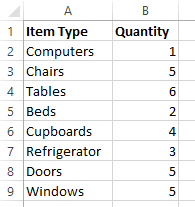You can use =COUNTIF(B2:B9,B9) or =COUNTIF(B2:B9,5) for this counting.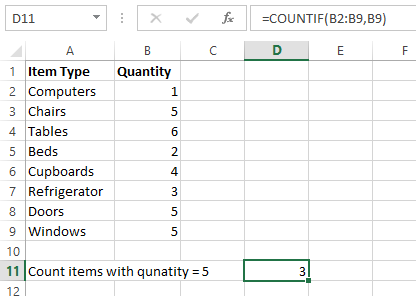Similarly, this function can be used to count cells with contents. See the next example.

• Consider the following data set and you want to count number of Mondays.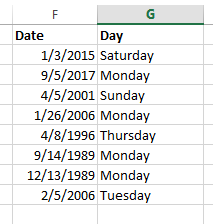You can use =COUNTIF(G2:G9,"Monday") or =COUNTIF(G2:G9,G8) for this counting. Note that when you are using a text condition, you have to type it within double quotation marks. Another thing that you better remember is criteria is case insensitive. Therefore though you use =COUNTIF(G2:G9,"monday") it will give you the same result.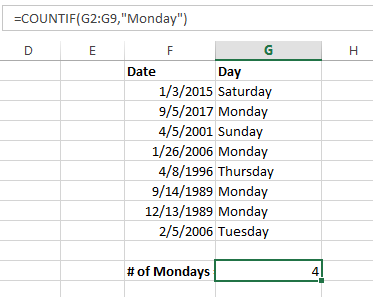Think that you want to count Saturdays and Sundays, you can still use this function. Since both starts with “S” we can use a simple syntax.

• =COUNTIF(G2:G9, "S*")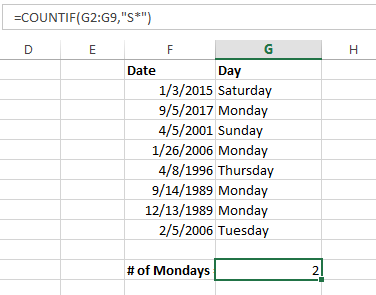• Note: An asterisk (*) is used to find cell contents with any sequence of leading and trailing characters. If you want to find any single character you can use a question mark (?)

As you can see this function can be used for many applications with different criteria. The following table contains some of the most used applications of COUNTIF() function.

 Condition/ Criteria Description =COUNTIF(range, "*nd*") Counts texts with “nd” anywhere within the cell =COUNTIF(range, "???day") Counts cells with exactly six characters ending with “day”. =COUNTIF(range,"*") Counts all cells that contain any text, however cells with dates and numbers will be considered as blank cells. =COUNTIF(range,"<>"&"") Counts all cells that contain any text, dates, numbers =COUNTIF(range,"") Counts all blank cells =COUNTIF(range,">5") Counts cells where value is greater than 5 =COUNTIF(range,">=6/21/2016") Counts cells with a date greater than or equal to 6/1/2014.

Tip: To count cells containing an actual question mark or asterisk, type a tilde () before the ? or * character in the criteria. (e.g. =COUNTIF(range,"*?*")